• 学生成绩管理系统总结
2021-05-19 20:43:16

《c语言下学生成绩管理系统程序设计报告》由会员分享，可在线阅读，更多相关《c语言下学生成绩管理系统程序设计报告(17页珍藏版)》请在人人文库网上搜索。

1、信息技术学院本科课程设计报告课程设计题目学 生 姓 名： 黄海兰 学 院： 信息技术学院 专 业： 信息管理与信息系统 班 级： B11203 学 号： 指 导 教 师： 尹剑伟 完 成 日 期： 2013 .7.12 辽 东 学 院Eastern Liaoning University一、题目描述模拟学校学生成绩管理系统。设计一个简单的学生成绩管理系统，实现对成绩管理的各种信息进行日常管理，如查询、修改、排序、增加、删除。二、功能分析通过体验并分析后得出中主要功能包括：1) 学生信息输入2) 学生信息输出3) 按照学号查询4) 学生信息修改5) 学生成绩排序6) 学生数据导入7) 学生数据保。

2、存8) 推出系统三、系统设计1程序总体结构程序中由主模块负责协调各功能模块的工作。运行程序成功后，根据输入操作，执行相应的输入、输出、查询、修改。排序、导入、保存、退出等功能。图1 学生成绩管理系统结构图2界面设计如图2，运行程序，然后输入密码进入学生成绩管理系统。图2 学生成绩管理的界面3输入方式设计此系统为dos下程序，因此简化为键盘控制。主要用到字母键，数字键，空格，退格，回车。搜狗拼音输入汉字。4重要数据的数据结构设计1) 全局变量int choice;int check=0;int loop=0;int a=12;2) 宏定义#define N 33) 结构体struct grade。

3、float math;/数学float chinese;/语文float english;/英语float computer;/计算机struct studentint num;/学号char name7;/姓名char sex3;/性别int age;/年龄struct grade score;struct student studN;5函数设计文件中函数的功能如下：文件名函数原形 函数功能函数处理描述学生成绩管理系统.cvoid denglu();登陆函数；用for语句控制密码输入次数。void xuanze();选择要进入的选项；输入序号选择进入模块；void inputinfo();输。

4、入学生信息；用if语句判断能否继续输入学生信息，根据提示输入学生信息及各科成绩；void outputinfo();输出学生信息用for循环输出学生基本信息、各科成绩和平均成绩；void find();按学号查询学生信息；利用for循环进行查找，用if 语句判断是否找到要找的信息，然后显示。void sortscore();按平均成绩对学生信息进行排序；首先输入信息，然后利用排序子函数进行排序从而实现插；void modify();创建学生信息；利用for循环把要删除的结构体变量用后面的覆盖，且从此处以后后面的变量覆盖前面的，以此完成删除任务；void writetofile();按姓名删除学。

6、 每10个暂停显示数据 5、回到主菜单，输入3，回车 出现排序成功信息。 6、回到主菜单，输入4，回车 按提示插入一组数据 7、回到主菜单，输入5，回车 按提示输入姓名，删除数据 出现删除成功的信息 8、回到主菜单，输入6，回车 输入姓名进行查询 9、回到主菜单，输入7，回车 出现统计信息 10、回到主菜单，输入9，回车 输入result.txt，回车 出现成功写入文件的信息 11、回到主菜单，输入0，回车退出系统六、总结通过这次课程设计的学习，我对c语言有了更深的认识。作为一门语言他也能根据我们的需要编写出相应的程序。在不断地修改、调试和添加中我对程序的结构和层次方面有了进一步的了解，这次学。

7、习让我深知程序设计不是想象中的那么简单，能够准确灵活的运用c语言我还得再下一些苦功夫。附一 使用说明：功能说明. 此程序分为8个选项，输入，输出、查询、修改、排序、退出等几个部分，进入1程序后，按提示输入学生信息；进入2程序后，输出1输入的学生信息；进入程序3后，输入学号，查找出学生信息；进入程序4后，输入学号修改学生信息；进入程序5后，对学生成绩排序；进入6后，进行学生数据导入；进入程序7后，保存已修改学生信息。附2、代码清单/*程序设计语言课程设计题目：学生成绩管理系统制作人：黄海兰完成日期：2012-7-11版本号：*/以下为文件包含#include #include #include 。

8、/宏定义#define N 3/以下为函数提前声明void denglu(int a);void xuanze();void inputInfo(); void outputInfo();void find();void sortscore();void modify();void writetofile();void readfile();/以下是全局变量int choice;int check=0;int loop=0;int a=12;/*类型：结构体名称：grade用途：描述学生成绩信息*/struct gradefloat math;/数学float chinese;/语文float。

9、 english;/英语float computer;/计算机float ave;/平均成绩;/*类型：结构体名称：student用途：描述学生基本信息*/struct studentint num;/学号char name7;/姓名char sex3;/性别int age;/年龄struct grade score;struct student studN;/*类型：函数名称：main用途：主函数形参：无返回值：无*/void main()printf(-n);printf(*欢迎使用学生成绩管理系统* n); printf(*技术支持 黄海兰* n );printf(*联系方式QQ* * 。

10、n );printf(*01256 65* n);printf(-n);denglu(a);readfile();system(cls); while(1) xuanze(); scanf(%d,&choice);switch(choice)case 1: inputInfo();break; case 2: if(loop=0)printf(没有输入或导入有效数据!n);outputInfo(); break; case 3: find(); break;case 4: modify(); break;case 5: sortscore();break;case 6: printf(警告!导。

11、入数据将覆盖现有未保存的数据n);printf(要继续吗?(输入1继续,输入0取消操作):);int r;scanf(%d,&r);if(r=1)readfile();break;elsebreak;case 7: writetofile();if(check=loop)printf(*数据输出成功*n);elseprintf(*数据输出失败*n);break;case 8: exit(0);default: printf(请正确选择服务项目n);/*类型：函数名称：denglu用途：登陆函数形参：a为进入此程序的密码返回值：无*/void denglu(int a)int mima,count=0;for(int i=0;istudk+1.score.ave)temp=studk;studk=studk+1;studk+1=temp;printf (学生成绩由高到低依次是:n);printf (n);for (j=0;jk;j+)printf (第%d名:%s 成绩:%fn,j+1,studloop.name,studloop.score.ave);printf (n。

更多相关内容
• 1.学生成绩管理系统分为三个用户角色，登录界面可以选择三种不同的角色进行登录系统 2.管理员角色登录系统可以有以下功能： 管理考试信息：可以对考试批次信息进行增删改查操作 管理教师信息：可以对教师个人信息...
• C 语言课程设计学生成绩管理系统 个人心得体会 徐州工程学院 14 计嵌 1 班 07 组 张凯 这一周以来的课程设计不仅让我明白了 C 语言的确是一门很有用的但是又是不容 易的一门课程 起初刚开始做课程设计时 以为这个...
• ## c语言学生成绩管理系统总结

千次阅读 多人点赞 2020-12-27 11:09:03
一、系统功能 1.基本功能 1.录入信息 2.按照学号查找信息 3.按照学号修改信息 4.按照学号删除信息 5.插入信息 6.浏览全部信息 7.按c语言成绩排序 8.保存到文件 2.结构体 typedef struct student { char num; /...

c语言的大作业，主要功能是录入、修改、删除、插入、排序等，可能有些地方仍然有点bug。

一、系统功能
1.基本功能

1.录入信息
2.按照学号查找信息
3.按照学号修改信息
4.按照学号删除信息
5.插入信息
6.浏览全部信息
7.按c语言成绩排序
8.保存到文件


2.结构体
只写了几个基础的，学号刚开始写的int型的，但后来不知道怎么去查重等，改成了char型。

typedef struct student
{
char num;     //学号
char name;    //姓名
char sex;     //性别
char duty;    //专业
int eng;          //英语成绩
int math;         //数学成绩
int c;            //c语言成绩
struct student *next;next是指针变量，指向结构体变量
}stu;


3.main函数

int main()
{
system("color 3F");//背景颜色
while(1)
{
printf("\t\t\t\t**********新生管理系统************\n");
printf("\t\t\t\t请选择你的操作\n");
printf("\t\t\t\t1.录入信息\n");
printf("\t\t\t\t2.按照学号查找信息\n");
printf("\t\t\t\t3.按照学号修改信息\n");
printf("\t\t\t\t4.按照学号删除信息\n");
printf("\t\t\t\t5.插入信息\n");
printf("\t\t\t\t6.浏览全部信息\n");
printf("\t\t\t\t7.按c语言成绩排序\n");
printf("\t\t\t\t8.保存到文件\n");
printf("\t\t\t\t0.退出\n");
printf("\t\t\t\t**********************************\n");
printf("\t\t\t\t请按键选择操作：\n");
fflush(stdin);//清除多余的未被保存的数据，防止乱码
int c;
scanf("%d",&c);
switch(c)//用switch语句进行选择和调用函数
{
case 1:
break;
case 2:
break;
case 3:
break;
case 4:
break;
case 5:
break;
case 6:
break;
case 7:
break;
case 8:
break;
case 0:
return 0;
default:
printf("输入错误!\n");
}
}
}


while循环在外，系统程序能够持续运行，switch判断语句进行功能选择与函数切换。

color[attr]
attr        指定控制台输出的颜色属性。
颜色属性由两个十六进制数字指定
第一个对应于背景，第二个对应于前景：
0 = 黑色              8 = 灰色
1 = 蓝色              9 = 淡蓝色
2 = 绿色              A = 淡绿色
3 = 浅绿色            B = 淡浅绿色
4 = 红色              C = 淡红色
5 = 紫色              D = 淡紫色
6 = 黄色              E = 淡黄色
7 = 白色              F = 亮白色


二、录入学生信息
在第一次验课设的时候，只简单地写了录入，没有写查重以及格式等的限制。在最后一次验的时候，学号查重依然有问题，遍历时应注意不能直接动头指针p，使q2=p，用q2实现遍历。

void input(stu *p)//录入
{
int n,x;
system("cls");   //清屏函数
stu *q1,*q2;     //定义该结构体类型q1,q2指针
q1=p;

printf("请输入要录入的学生人数：");
scanf("%d",&n);
while(n--)  //while循环n次，n个要录入的学生
{
stu *q=(stu *)malloc(sizeof(stu)); //给q分配内存

int i=1; //定义i循环三次使学号只能输入数字
int flag=1,flag1=1,flag2=1,flag3=1,flag4=1;
loop1:while(flag)
{
printf("请输入学号(三位):");
scanf("%s",q->num);
if(strlen(q->num)!=3)//用strlen函数计算字符串长度使学号为三位数
{
printf("学号格式输入错误!\n");
fflush(stdin);//清除多余的未被保存的数据，防止乱码
continue;
}
q2=p; //头指针赋给q2
while(q2)
{
if(strcmp(q->num,q2->num)==0)//用strcmp函数，q2遍历查重
{
printf("输入的学号重复,请重新输入!\n");
goto loop1;//重复重新跳到上一个while循环
}
q2=q2->next;
}
while(i<3)
{
if(q->num[i]-'0'<0 || q->num[i]-'0'>9)//让学号只能输入数字
{
printf("学号格式输入错误!\n");
break;
}
else{
i++;
if (i==3)
flag=0;
}
}
}
printf("请输入学生姓名:");
scanf("%s",q->name);

while(flag1)
{
printf("请输入性别（w/m):");
scanf("%s",q->sex);
if(strcmp(q->sex,"w")==0)//用strcmp函数限制输入的字符，只能输入w/m
flag1=0;//跳出循环
else if(strcmp(q->sex,"m")==0)
flag1=0;//跳出循环
else
{
printf("性别格式输入错误!(请输入w/m)\n");
}
}
printf("请输入专业:");
scanf("%s",q->duty);

while (flag2)
{
printf("请输入英语成绩(0<=eng<=150):");
x=scanf("%d",&q->eng); //如果输入数字x=1
if(q->eng >= 0 && q->eng <= 150 && x==1 )//限制成绩范围
flag2=0;//跳出循环
else{
printf("成绩格式输入错误!\n");
fflush(stdin);//清理未被保存的数据
}
}

while(flag3)
{
printf("请输入数学成绩(0<=math<=150):");
x=scanf("%d",&q->math);
if(q->math>=0 && q->math<=150 && x==1)
flag3=0;
else{
printf("成绩格式输入错误!\n");
fflush(stdin);
flag3=1;
}
}

while(flag4){
printf("请输入c语言成绩(0<=c<=150):");
x=scanf("%d",&q->c);
if(q->c>=0 && q->c<=150 && x==1)
flag4=0;
else{
printf("成绩格式输入错误!\n");
fflush(stdin);
flag4=1;
}
}
printf("录入成功!\n");
q->next=NULL; //使q中的next定义为空
q1->next=q;
q1=q;
}
}


三、查找学生信息

void seek(stu *p)//查找
{
stu *q=p->next;
char num;
int flag = 0;
system("cls"); 	//清屏函数
printf("请输入查找学生的学号：");
scanf("%s",num);
while(q!=NULL)
{
if(strcmp(q->num,num) == 0)//strcmp函数查找学生学号
{
printf("学生学号：%s\n",q->num);
printf("学生姓名：%s\n",q->name);
printf("学生性别：%s\n",q->sex);
printf("专业：%s\n",q->duty);
printf("英语成绩：%d\n",q->eng);
printf("数学成绩：%d\n",q->math);
printf("c语言成绩：%d\n",q->c);
flag = 1;
break;
}
q=q->next;//遍历
}
if(flag == 0)
{
printf("查无此人\n");
}
}


四、按照学号修改学生信息
在录入函数源代码的基础上写了修改功能。

void revise(stu *p)//修改
{
stu *q=p->next;
stu *q1,*q2;
int x;
char num;
system("cls");
printf("请输入要修改的学号:");
scanf("%s",num);
while(q!=NULL)
{
if(strcmp(q->num,num) == 0)
{
int i=1;
int flag=1,flag1=1,flag2=1,flag3=1,flag4=1;
loop1:while(flag)
{
printf("请输入学号(三位):");
scanf("%s",q->num);
if(strlen(q->num)!=3){
printf("学号格式输入错误!\n");
fflush(stdin);
continue;
}
q2=p;
while(q2)
{
if(strcmp(q->num,q2->num)==0)
{
printf("输入的学号重复,请重新输入!\n");
goto loop1;
}
else{
break;
}
q2=q2->next;
}
while(i<3)
{
if(q->num[i]-'0'<0 || q->num[i]-'0'>9){
printf("学号格式输入错误!\n");
break;
}
else{
i++;
if (i==3)
flag=0;
}
}
}
printf("请输入学生姓名:");
scanf("%s",q->name);

while(flag1)
{
printf("请输入性别（w/m):");
scanf("%s",q->sex);
if(strcmp(q->sex,"w")==0)
flag1=0;
else if(strcmp(q->sex,"m")==0)
flag1=0;
else
{
printf("性别格式输入错误!(请输入w/m)\n");
}
}
printf("请输入专业:");
scanf("%s",q->duty);

while (flag2)
{
printf("请输入英语成绩(0<=eng<=150):");
x=scanf("%d",&q->eng);
if(q->eng >= 0 && q->eng <= 150 && x==1 )
flag2=0;
else{
printf("成绩格式输入错误!\n");
fflush(stdin);
}
}

while(flag3)
{
printf("请输入数学成绩(0<=math<=150):");
x=scanf("%d",&q->math);
if(q->math>=0 && q->math<=150 && x==1)
flag3=0;
else{
printf("成绩格式输入错误!\n");
fflush(stdin);
flag3=1;
}
}

while(flag4){
printf("请输入c语言成绩(0<=c<=150):");
x=scanf("%d",&q->c);
if(q->c>=0 && q->c<=150 && x==1)
flag4=0;
else{
printf("成绩格式输入错误!\n");
fflush(stdin);
flag4=1;
}
}
printf("修改成功!\n");
return;
}
q=q->next;
q2->next=q;
q2=q;
}
printf("查无此人");
}


五、按照学号删除信息
注意while(!strcmp(q2->num,num))，刚开始写时while(strcmp(q2->num,num))一直删除不成功，当q2->num和num相等时为0，while循环无法进行。

void del(stu *p)//删除
{
stu *q1,*q2;//定义该结构体类型q1,q2指针
q1=p; //q1头结点，p头指针
q2=q1->next;
char num;
system("cls");
printf("请输入想要删除的学号:");
scanf("%s",num);
while(q2!=0)
{
while(!strcmp(q2->num,num))
{
q1->next=q2->next;
free(q2);//释放q2
q2=NULL;
printf("删除成功\n");
return;
}
q1=q2;
q2=q2->next;
}
}


六、插入信息（头插法）
插入部分也是结合的头插法和录入函数

void add(stu *p) //插入
{
int n,x;
int i=1;
int flag=1,flag1=1,flag2=1,flag3=1,flag4=1;
stu *q,*q1,*q2;//不用像尾插法一样生成一个终端结点
q=(stu*)malloc(sizeof(stu));//给q分配内存
q1=(stu*)malloc(sizeof(stu));//给q1分配内存
q = p;//q为头结点
if(q1==NULL)
{
printf("插入失败\n");
exit(1);
}
for(int a=0;a<n;a++);
{

loop1:while(flag)
{
printf("请输入学号(三位):");
scanf("%s",q1->num);
if(strlen(q1->num)!=3){
printf("学号格式输入错误!\n");
fflush(stdin);
continue;
}
q2=p;
while(q2)
{
if(strcmp(q1->num,q2->num)==0)
{
printf("输入的学号重复,请重新输入!\n");
goto loop1;
}
q2=q2->next;
}
while(i<3)
{
if(q1->num[i]-'0'<0 || q1->num[i]-'0'>9){
printf("学号格式输入错误!\n");
break;
}
else{
i++;
if (i==3)
flag=0;
}
}
}
printf("请输入学生姓名:");
scanf("%s",q1->name);

while(flag1)
{
printf("请输入性别（w/m):");
scanf("%s",q1->sex);
if(strcmp(q1->sex,"w")==0)
flag1=0;
else if(strcmp(q1->sex,"m")==0)
flag1=0;
else
{
printf("性别格式输入错误!(请输入w/m)\n");
}
}
printf("请输入专业:");
scanf("%s",q1->duty);

while (flag2)
{
printf("请输入英语成绩(0<=eng<=150):");
x=scanf("%d",&q1->eng);
if(q1->eng >= 0 && q1->eng <= 150 &&x==1 )
flag2=0;
else{
printf("成绩格式输入错误!\n");
fflush(stdin);
}
}

while(flag3)
{
printf("请输入数学成绩(0<=math<=150):");
x=scanf("%d",&q1->math);
if(q1->math>=0 && q1->math<=150 && x==1)
flag3=0;
else{
printf("成绩格式输入错误!\n");
fflush(stdin);
flag3=1;
}
}

while(flag4){
printf("请输入c语言成绩(0<=c<=150):");
x=scanf("%d",&q1->c);
if(q1->c>=0 && q1->c<=150 && x==1)
flag4=0;
else{
printf("成绩格式输入错误!\n");
fflush(stdin);
flag4=1;
}
}
q1->next=p->next;将p指向的地址赋值给q1;
p->next=q1;头指针的指针域next指向q1节点，使得q1成为开始节点
q1=q1->next;
printf("插入成功！\n");
}
}七、浏览全部信息

void traver(stu *p) //遍历输出
{
stu *q = p->next ;//使p的next给q
system("cls"); 	//清屏
while(q!=NULL)
{
printf("学号:%s\n姓名:%s\n性别:%s\n专业:%s\n英语成绩:%d\n数学成绩:%d\nc语言成绩:%d\n",q->num,q->name,q->sex,q->duty,q->eng,q->math,q->c);
q=q->next;
}
}


八、按c语言成绩排序（降序）

void sort(stu *p)//排序 (c语言成绩）
{
stu *q=NULL;
system("cls");
while(q!=p->next) //q不等于头指针是一次循环
{
stu *pre=p;
stu *cur=pre->next;
while(cur!=q&&cur->next !=q)
{
if(cur->c<cur->next->c )
{
pre->next =cur->next;
cur->next =cur->next->next;
pre->next->next =cur;
}
pre=pre->next;
cur=pre->next;
}
q=cur;
}
traver(p);//调用traver函数输出结果
}


九、保存文件

void save(stu *p)//文件
{
FILE*fp = fopen("学生信息.txt","w");//创建文件指针
if(fp!=NULL)
{

while(p->next!=NULL)
{
fprintf(fp,"学号：%s\n",p->next->num);
fprintf(fp,"姓名：%s\n",p->next->name);
fprintf(fp,"性别：%s\n",p->next->sex);
fprintf(fp,"专业：%s\n",p->next->duty);
fprintf(fp,"英语成绩：%d\n",p->next->eng);
fprintf(fp,"数学成绩：%d\n",p->next->math);
fprintf(fp,"c语言成绩：%d\n",p->next->c);
fprintf(fp,"---------------------------\n");
p=p->next;
}
printf("保存成功！\n");
}
fclose(fp);
}


全部代码

#include<stdio.h>
#include<stdlib.h>
#include<string.h>
typedef struct student
{
char num;
char name;
char sex;
char duty;
int eng;
int math;
int c;
struct student *next;
}stu;

void input(stu *p)//录入
{
int n,x;
system("cls");
stu *q1,*q2;
q1=p;

printf("请输入要录入的学生人数：");
scanf("%d",&n);
while(n--)
{
stu *q=(stu *)malloc(sizeof(stu));

int i=1;
int flag=1,flag1=1,flag2=1,flag3=1,flag4=1;
loop1:while(flag)
{
printf("请输入学号(三位):");
scanf("%s",q->num);
if(strlen(q->num)!=3){
printf("学号格式输入错误!\n");
fflush(stdin);
continue;
}
q2=p;
while(q2)
{
if(strcmp(q->num,q2->num)==0)
{
printf("输入的学号重复,请重新输入!\n");
goto loop1;
}
q2=q2->next;
}
while(i<3)
{
if(q->num[i]-'0'<0 || q->num[i]-'0'>9){
printf("学号格式输入错误!\n");
break;
}
else{
i++;
if (i==3)
flag=0;
}
}
}
printf("请输入学生姓名:");
scanf("%s",q->name);

while(flag1)
{
printf("请输入性别（w/m):");
scanf("%s",q->sex);
if(strcmp(q->sex,"w")==0)
flag1=0;
else if(strcmp(q->sex,"m")==0)
flag1=0;
else
{
printf("性别格式输入错误!(请输入w/m)\n");
}
}
printf("请输入专业:");
scanf("%s",q->duty);

while (flag2)
{
printf("请输入英语成绩(0<=eng<=150):");
x=scanf("%d",&q->eng);
if(q->eng >= 0 && q->eng <= 150 && x==1 )
flag2=0;
else{
printf("成绩格式输入错误!\n");
fflush(stdin);
}
}

while(flag3)
{
printf("请输入数学成绩(0<=math<=150):");
x=scanf("%d",&q->math);
if(q->math>=0 && q->math<=150 && x==1)
flag3=0;
else{
printf("成绩格式输入错误!\n");
fflush(stdin);
flag3=1;
}
}

while(flag4){
printf("请输入c语言成绩(0<=c<=150):");
x=scanf("%d",&q->c);
if(q->c>=0 && q->c<=150 && x==1)
flag4=0;
else{
printf("成绩格式输入错误!\n");
fflush(stdin);
flag4=1;
}
}
printf("录入成功!\n");
q->next=NULL;
q1->next=q;
q1=q;
}
}

void traver(stu *p) //遍历输出
{
stu *q = p->next ;
system("cls");
while(q!=NULL)
{
printf("学号:%s\n姓名:%s\n性别:%s\n专业:%s\n英语成绩:%d\n数学成绩:%d\nc语言成绩:%d\n",q->num,q->name,q->sex,q->duty,q->eng,q->math,q->c);
q=q->next;
}
}

{
int n,x;
int i=1;
int flag=1,flag1=1,flag2=1,flag3=1,flag4=1;
stu *q,*q1,*q2;
q=(stu*)malloc(sizeof(stu));
q1=(stu*)malloc(sizeof(stu));
q = p;
if(q1==NULL)
{
printf("插入失败\n");
exit(1);
}
for(int a=0;a<n;a++);
{

loop1:while(flag)
{
printf("请输入学号(三位):");
scanf("%s",q1->num);
if(strlen(q1->num)!=3){
printf("学号格式输入错误!\n");
fflush(stdin);
continue;
}
q2=p;
while(q2)
{
if(strcmp(q1->num,q2->num)==0)
{
printf("输入的学号重复,请重新输入!\n");
goto loop1;
}
q2=q2->next;
}
while(i<3)
{
if(q1->num[i]-'0'<0 || q1->num[i]-'0'>9){
printf("学号格式输入错误!\n");
break;
}
else{
i++;
if (i==3)
flag=0;
}
}
}
printf("请输入学生姓名:");
scanf("%s",q1->name);

while(flag1)
{
printf("请输入性别（w/m):");
scanf("%s",q1->sex);
if(strcmp(q1->sex,"w")==0)
flag1=0;
else if(strcmp(q1->sex,"m")==0)
flag1=0;
else
{
printf("性别格式输入错误!(请输入w/m)\n");
}
}
printf("请输入专业:");
scanf("%s",q1->duty);

while (flag2)
{
printf("请输入英语成绩(0<=eng<=150):");
x=scanf("%d",&q1->eng);
if(q1->eng >= 0 && q1->eng <= 150 &&x==1 )
flag2=0;
else{
printf("成绩格式输入错误!\n");
fflush(stdin);
}
}

while(flag3)
{
printf("请输入数学成绩(0<=math<=150):");
x=scanf("%d",&q1->math);
if(q1->math>=0 && q1->math<=150 && x==1)
flag3=0;
else{
printf("成绩格式输入错误!\n");
fflush(stdin);
flag3=1;
}
}

while(flag4){
printf("请输入c语言成绩(0<=c<=150):");
x=scanf("%d",&q1->c);
if(q1->c>=0 && q1->c<=150 && x==1)
flag4=0;
else{
printf("成绩格式输入错误!\n");
fflush(stdin);
flag4=1;
}
}
q1->next=p->next;
p->next=q1;
q1=q1->next;
printf("插入成功！\n");
}
}

void seek(stu *p)//查找
{
stu *q=p->next;
char num;
int flag = 0;
system("cls");
printf("请输入查找学生的学号：");
scanf("%s",num);
while(q!=NULL)
{
if(strcmp(q->num,num) == 0)
{
printf("学生学号：%s\n",q->num);
printf("学生姓名：%s\n",q->name);
printf("学生性别：%s\n",q->sex);
printf("专业：%s\n",q->duty);
printf("英语成绩：%d\n",q->eng);
printf("数学成绩：%d\n",q->math);
printf("c语言成绩：%d\n",q->c);
flag = 1;
break;
}
q=q->next;
}
if(flag == 0)
{
printf("查无此人\n");
}
}

void revise(stu *p)//修改
{
stu *q=p->next;
stu *q1,*q2;
int x;
char num;
system("cls");
printf("请输入要修改的学号:");
scanf("%s",num);
while(q!=NULL)
{
if(strcmp(q->num,num) == 0)
{
int i=1;
int flag=1,flag1=1,flag2=1,flag3=1,flag4=1;
loop1:while(flag)
{
printf("请输入学号(三位):");
scanf("%s",q->num);
if(strlen(q->num)!=3){
printf("学号格式输入错误!\n");
fflush(stdin);
continue;
}
q2=p;
while(q2)
{
if(strcmp(q->num,q2->num)==0)
{
printf("输入的学号重复,请重新输入!\n");
goto loop1;
}
else{
break;
}
q2=q2->next;
}
while(i<3)
{
if(q->num[i]-'0'<0 || q->num[i]-'0'>9){
printf("学号格式输入错误!\n");
break;
}
else{
i++;
if (i==3)
flag=0;
}
}
}
printf("请输入学生姓名:");
scanf("%s",q->name);

while(flag1)
{
printf("请输入性别（w/m):");
scanf("%s",q->sex);
if(strcmp(q->sex,"w")==0)
flag1=0;
else if(strcmp(q->sex,"m")==0)
flag1=0;
else
{
printf("性别格式输入错误!(请输入w/m)\n");
}
}
printf("请输入专业:");
scanf("%s",q->duty);

while (flag2)
{
printf("请输入英语成绩(0<=eng<=150):");
x=scanf("%d",&q->eng);
if(q->eng >= 0 && q->eng <= 150 && x==1 )
flag2=0;
else{
printf("成绩格式输入错误!\n");
fflush(stdin);
}
}

while(flag3)
{
printf("请输入数学成绩(0<=math<=150):");
x=scanf("%d",&q->math);
if(q->math>=0 && q->math<=150 && x==1)
flag3=0;
else{
printf("成绩格式输入错误!\n");
fflush(stdin);
flag3=1;
}
}

while(flag4){
printf("请输入c语言成绩(0<=c<=150):");
x=scanf("%d",&q->c);
if(q->c>=0 && q->c<=150 && x==1)
flag4=0;
else{
printf("成绩格式输入错误!\n");
fflush(stdin);
flag4=1;
}
}
printf("修改成功!\n");
return;
}
q=q->next;
q2->next=q;
q2=q;
}
printf("查无此人");
}

void del(stu *p)//删除
{
stu *q1,*q2;
q1=p;
q2=q1->next;
char num;
system("cls");
printf("请输入想要删除的学号:");
scanf("%s",num);
while(q2!=0)
{
while(!strcmp(q2->num,num))
{
q1->next=q2->next;
free(q2);
q2=NULL;
printf("删除成功\n");
return;
}
q1=q2;
q2=q2->next;
}
}

void sort(stu *p)//排序 (c语言成绩）
{
stu *q=NULL;
system("cls");
while(q!=p->next)
{
stu *pre=p;
stu *cur=pre->next;
while(cur!=q&&cur->next !=q)
{
if(cur->c<cur->next->c )
{
pre->next =cur->next;
cur->next =cur->next->next;
pre->next->next =cur;
}
pre=pre->next;
cur=pre->next;
}
q=cur;
}
traver(p);
}

void save(stu *p)//文件
{
FILE*fp = fopen("学生信息.txt","w");
if(fp!=NULL)
{

while(p->next!=NULL)
{
fprintf(fp,"学号：%s\n",p->next->num);
fprintf(fp,"姓名：%s\n",p->next->name);
fprintf(fp,"性别：%s\n",p->next->sex);
fprintf(fp,"专业：%s\n",p->next->duty);
fprintf(fp,"英语成绩：%d\n",p->next->eng);
fprintf(fp,"数学成绩：%d\n",p->next->math);
fprintf(fp,"c语言成绩：%d\n",p->next->c);
fprintf(fp,"---------------------------\n");
p=p->next;
}
printf("保存成功！\n");
}
fclose(fp);
}

int main()
{
system("color 3F");
while(1)
{
printf("\t\t\t\t**********新生管理系统************\n");
printf("\t\t\t\t请选择你的操作\n");
printf("\t\t\t\t1.录入信息\n");
printf("\t\t\t\t2.按照学号查找信息\n");
printf("\t\t\t\t3.按照学号修改信息\n");
printf("\t\t\t\t4.按照学号删除信息\n");
printf("\t\t\t\t5.插入信息\n");
printf("\t\t\t\t6.浏览全部信息\n");
printf("\t\t\t\t7.按c语言成绩排序\n");
printf("\t\t\t\t8.保存到文件\n");
printf("\t\t\t\t0.退出\n");
printf("\t\t\t\t**********************************\n");
printf("\t\t\t\t请按键选择操作：\n");
fflush(stdin);
int c;
scanf("%d",&c);
switch(c)
{
case 1:
break;
case 2:
break;
case 3:
break;
case 4:
break;
case 5:
break;
case 6:
break;
case 7:
break;
case 8:
break;
case 0:
return 0;
default:
printf("输入错误!\n");
}
}
}

展开全文c语言
• Python学生成绩管理系统源码+.exe，Pycharm及自带IDLE完美运行，可用来应付大作业或作为基础学习，内含心得体会以及细节注释，易根据自身需要修改，源码在system文件夹里
• 学生成绩管理系统，开发软件Microsoft Visual Studio 2010，
• C 语言课程设计学生成绩管理系统 个人心得体会 徐州工程学院 14 计嵌 1 班 07 组 张凯 这一周以来的课程设计不仅让我明白了 C 语言的确是一门很有用的但是又是不容易的一门课程 起初刚开始做课程设计时 以为这个课程...
• 包括总体设计，界面设计，详细设计与实现，系统测试，设计总结等。适合参考模板。
• 学生成绩管理系统，分三个模块：学生，教师和管理员。 管理员模块：负责学生、老师信息的增删改；发布课程信息的增删改，以便让学生选课；审核老师提交的学生成绩并且打印成绩存档；按照课号查询每个课号的学生成绩...
• 5.管理员可查看所有学生的排名成绩和对指定学生查看排名成绩信息 6.管理员可查看所有学生某学科的平均分 7.管理员可查看多有学生某学科的及格人数和不及格人数 8.管理员可对某学科成绩信息进行数据备份 关于模块：...
• 该生按照课程考核模板要求，选取学习成绩管理系统进行了分析与设计。该系统主要面向教学管理用户，主要有学生查询、教师输入考试信息、辅导员查询导出学生成绩、教务处统计分析综合成等几个功能模块，基本功能已经...
• 数据库系统概论 课程设计 设计名称 学生信息管理系统数据库设计 指导老师 杨林 蔡亮 班级 学号 姓名 成绩 KT873-2 6 李龙 KT873-2 7 暴晋南 KT873-2 19 李琰 目 录 TOC \o "1-7" \h \z \u 一需求分析 2 1.1功能需求...
• 个人课程设计报告书（内含源码） 内含4个类，各自具有其属性和行为。 基本实现了： (1)学生基本信息、考试科目及成绩的信息录入...对学生成绩进行统计（包括最高分，最低分，排序，平均成绩，及格率和需要补考的学生）
• Python课程大作业代码参考 600行代码 实现功能满足大学课程基本要求 完全自我撰写 无参考无抄袭 满分作业 含总结报告
• 第一章 绪论 1.1 研究背景 1.2 研究现状 1.3 研究意义与目标 1.4 全文组织结构 ... 5.4.1 成绩功能实现 5.4.2 其他信息查询 5.5 管理员功能实现 5.6 本章小结 第六章 总结与展望 6.1 工作总结 6.2 研究展望
• 学生成绩管理系统（有3到4个程序 可以任选一个） 试编写一管理系统,要求如下: （1）实现对两个文件数据进行合并,生成新文件3.txt （2）抽取出三科成绩中有补考的学生并保存在一个新文件4.txt （3）对合并后的文件3....
• 计算机学院 C语言程序设计报告书 设计名称学生成绩管理系统 专业软件工程 指导老师 班级 姓名 学号 座号 2011-6-15 一实验目的 1.在编辑应用程序过程中逐步加深对C语言编程的理解 2.加强实践的能力动手能力同时...
• javawweb学生成绩管理系统实验报告，首先我做的系统是学生成绩管理系统使用jsp+javabean+servlet的传统的MVC开发模式，使用mysql数据库来存储数据。其中Jsp实现视图端，Javabean是模型端，Servlet是控制端。Servlet...
• 系统使用Visual Studio2017作为IDE，使用C++进行编写，其中排序功能使用了冒泡排序。包括源代码、文档报告、测试数据以及功能介绍文档。课程设计结束，给大家作为参考。
• 实训报告 实训名称 学生成绩管理系统 系部 专业班级 学生姓名 学 号 指导教师 完成日期 南京铁道职业技术学院 目 ? ? ? ? ? ? ? ? ? ? ? 装 ? ? ? ? ? ? ? ? ? ? ? ? ? ? ? ? ? ? ? ? ? ? ? ? ? ? ? ? ? ? ? ? ? ? ?...
• 明确用户对学生成绩管理系统的功能需求和性能需求，实现对学生成绩等数据进行有效管理，提供查询分析功能。总结软件开发过程中的方法和技巧，更好的应用和数据库技术 ，并将这些需求用规范化的语言和规范化的结构...软件工程 需求分析
• 本文实例为大家分享了shell实现学生成绩管理系统的具体代码，供大家参考，具体内容如下 基本的操作 增加 删除 查询 展示全部学生信息 vim编辑保存信息的文件 遇到的问题 1.增加学生信息时 增加学生信息时，我用的...
• 实训报告 实训名称 学生成绩管理系统 系 部 专业班级 学生姓名 学 号 指导教师 完成日期 南京铁道职业技术学院 ? 目 录 ? ? ? ? ? ? ? ? ? ? 装 ? ? ? ? ? ? ? ? ? ? ? ? ? ? ? ? ? ? ? ? ? ? ? ? ? ? ? ? ? ? ? ? ?...
• 本文采用php5.4+apache2.4，使用Mysql5.7数据库实现了网页版学生成绩管理系统，本系统分为三个模块：系统管理员模块、教师模块、学生模块。其中教师模块可以录入选课学生的成绩、修改成绩、查看成绩；学生模块可以...
• - - PAGE # - 目录 TOC \o "1-5" \h \z \o "Current Document" 摘要 - 2 - \o "Current Document" 系统开发目的 - 2 - \o "Current Document" 需求分析 - 3 - 总体功能需求 - 3 - 3.2. 各版块功能任务 - 5 - \o ...
• 精.选 目 录 TOC \o "1-3" \h \z \u 1 引言.2 3 需求分析.3 2.1 问题陈述 3 2.2 需求分析 4 2.3 可行性研究 4 3 系统总体设计 5 3.1 ...9 4.2.1 登录管理模块的实现 9 4.2.2 用户管理模块的实现 10 4.2.3 花卉管理
• 学生成绩管理系统，分三个模块：学生，教师和管理员。 管理员模块：负责学生、老师信息的增删改；发布课程信息的增删改，以便让学生选课；审核老师提交的学生成绩并且打印成绩存档；按照课号查询每个课号的学生成绩...php
• C语言大学课程作业 含课程报告 自我撰写 无抄袭无参考 满分 400行代码
• 基于完整javaEE学生信息管理系统文章结构一、开发框架及业务方向1.开发环境2.开发框架3.整体业务二、项目结构及页面展示1.项目整体结构2.学生页面3.教师页面4.管理员页面运行视频需要源码的加企鹅：671033846；备注...

### 基于ssm的学生成绩管理系统（信息，选课，考勤）# 文章结构

## 一、开发框架及业务方向

### 1.开发环境

• 操作系统不限：java特性，一套代码，导出运行
• jdk版本不限：推荐jdk1.8
• tomcat版本不限：推荐Tomcat8.0
• 数据库mysql：版本不限，推荐mysql8.0以下
• maven
• 开发工具：eclipse/idea 版本不限

### 2.开发框架

项目框架：ssm+mysql+wasyui+ajax+jquery

### 3.整体业务

本系统分为三种权限：
1.学生：考勤管理，请假，选课，查询成绩，修改个人信息等
2.教师：考勤管理，请假审核等，选课信息管理，学生信息管理，班级信息管理，
课程信息管理，成绩管理，成绩导入导出功能，成绩统计（可视化展示），修改个人信息等
3.管理员：比教师多一个教师管理

## 二、项目结构及页面展示

### 1.项目整体结构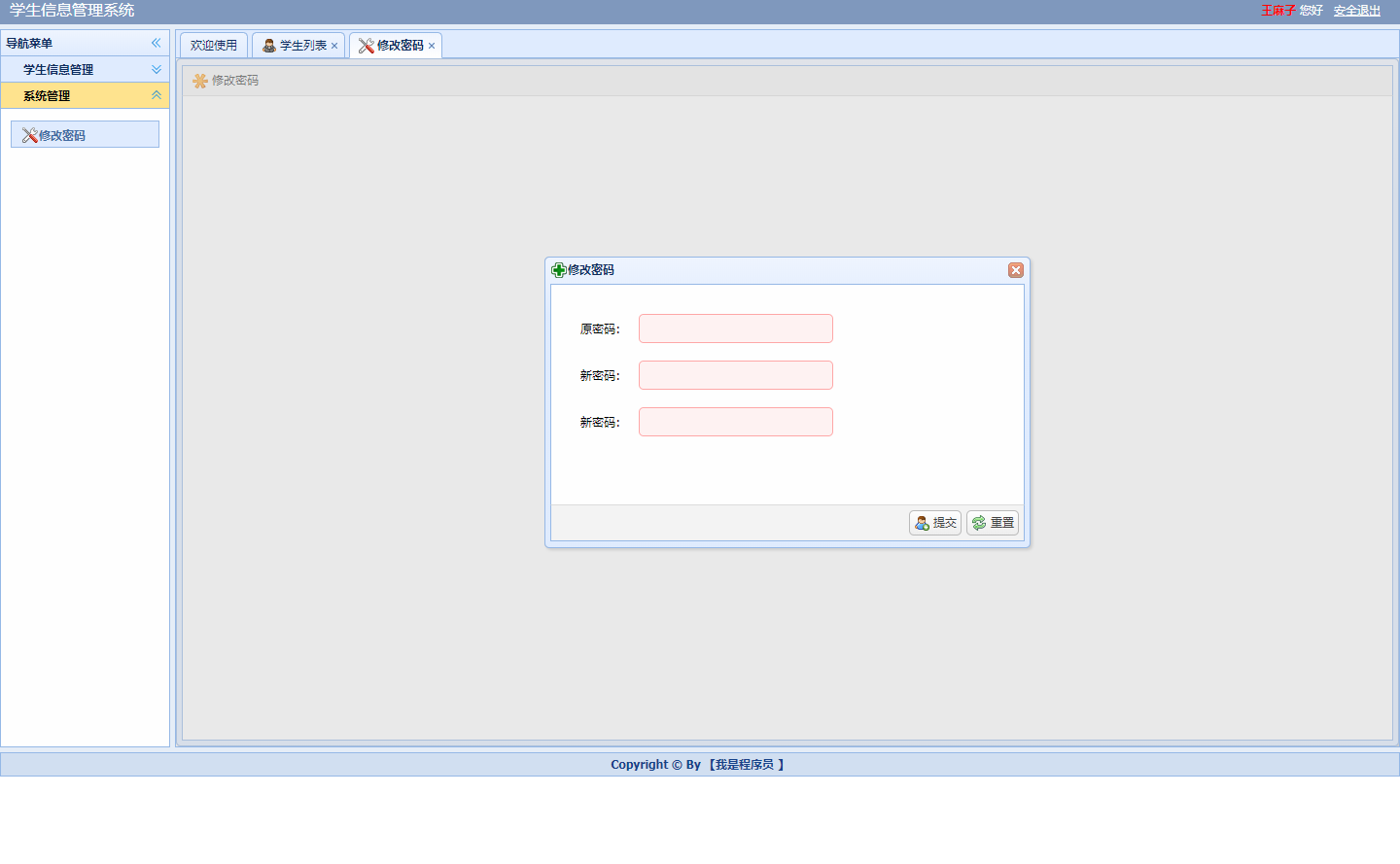### 2.学生页面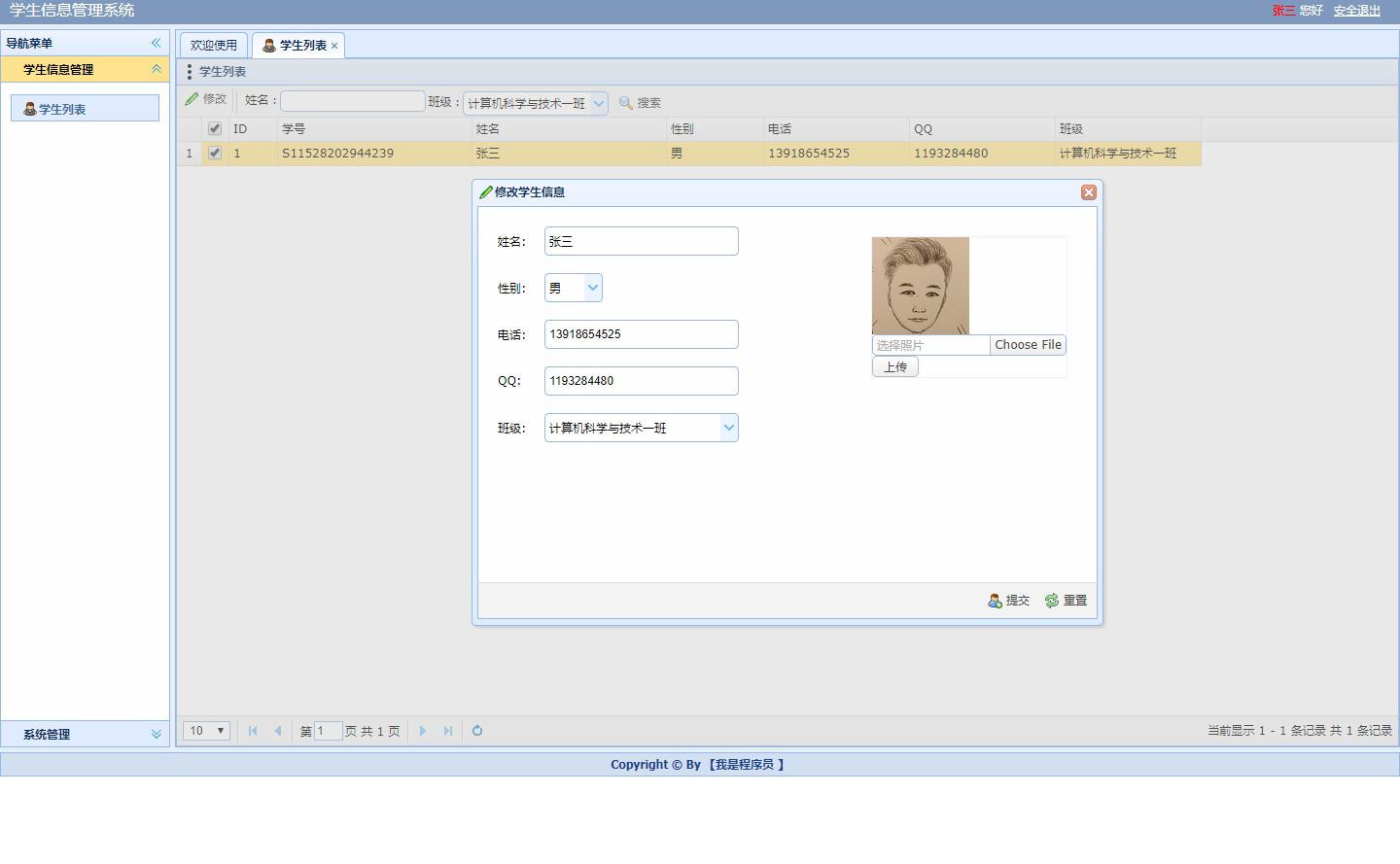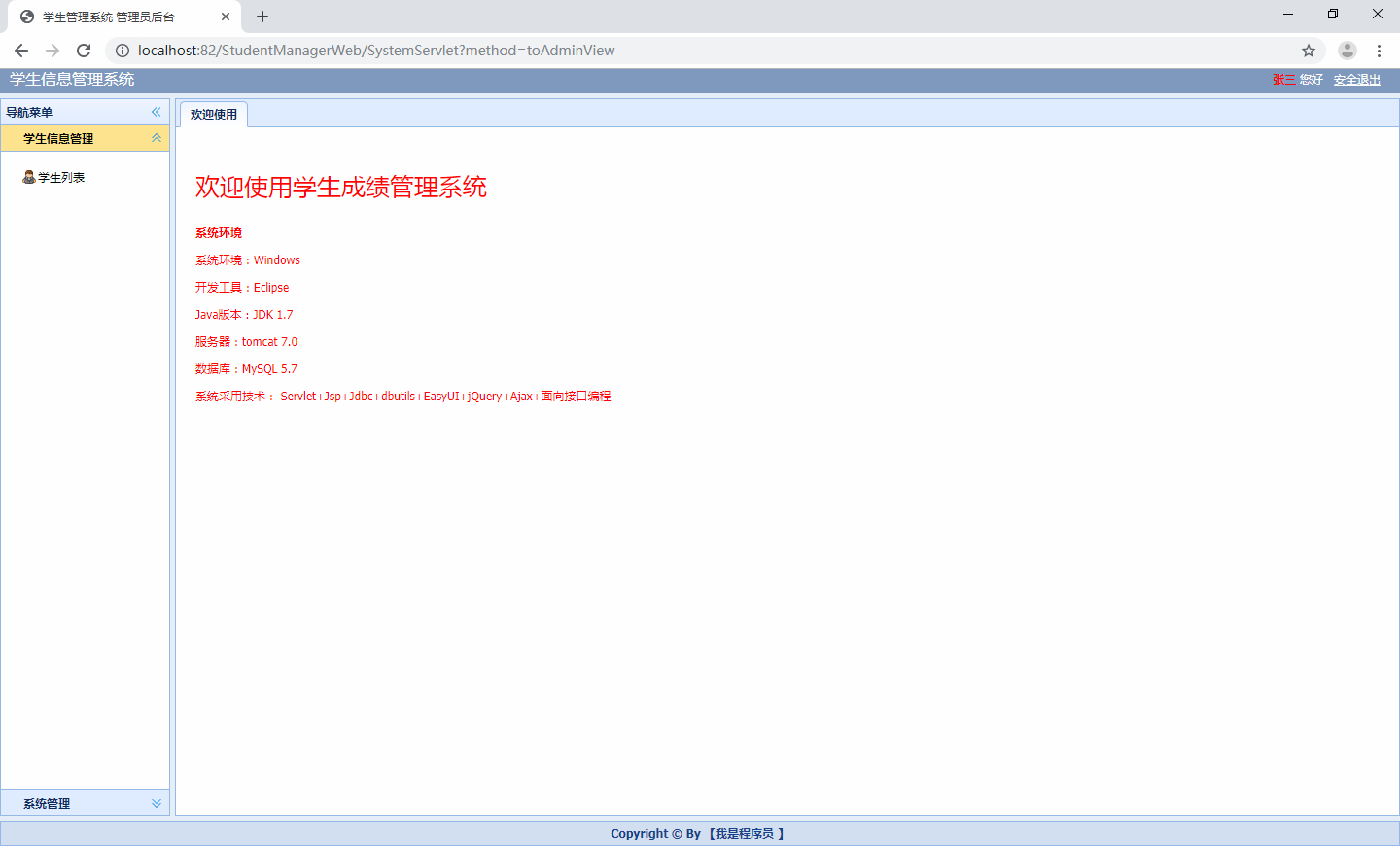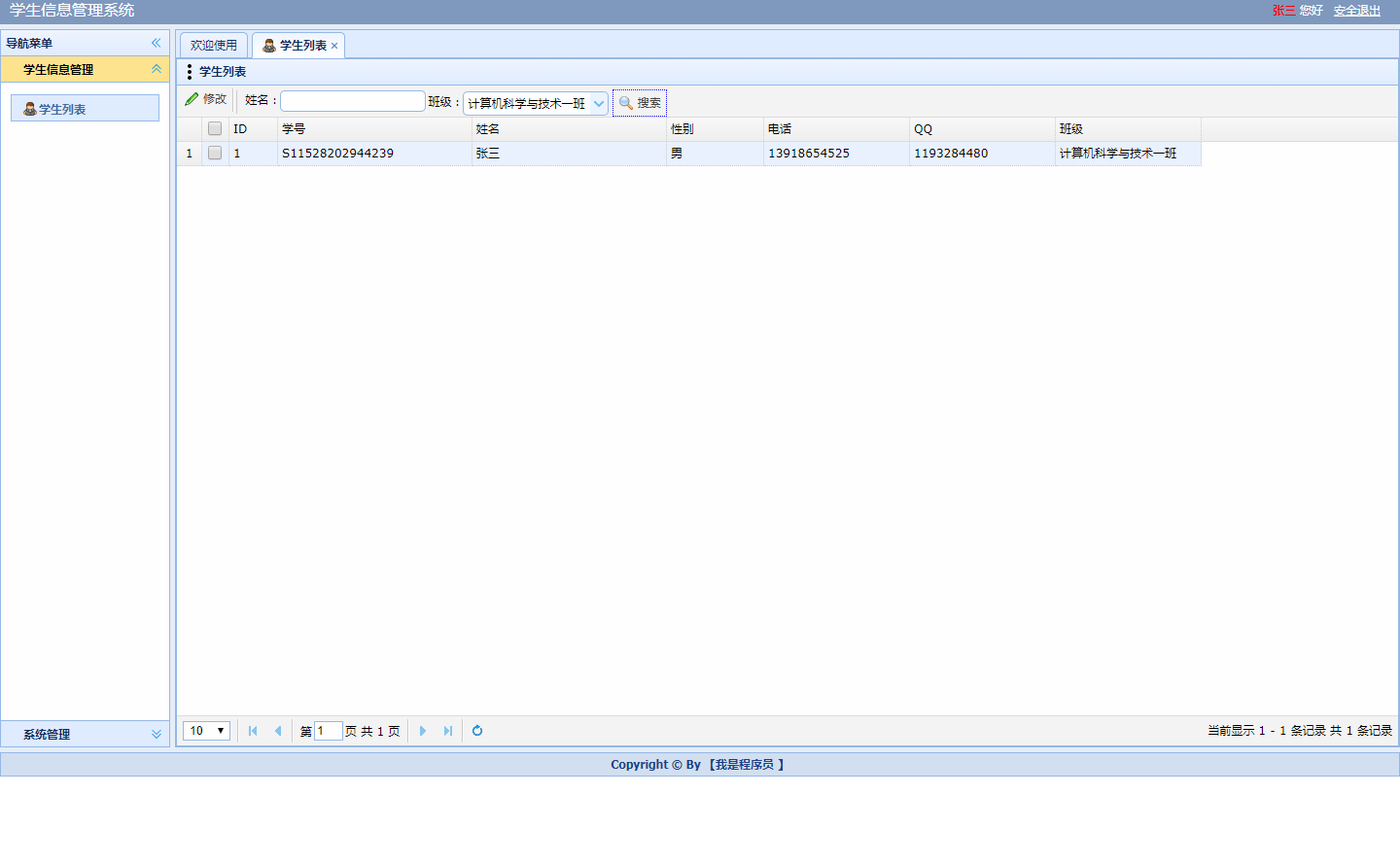### 3.教师页面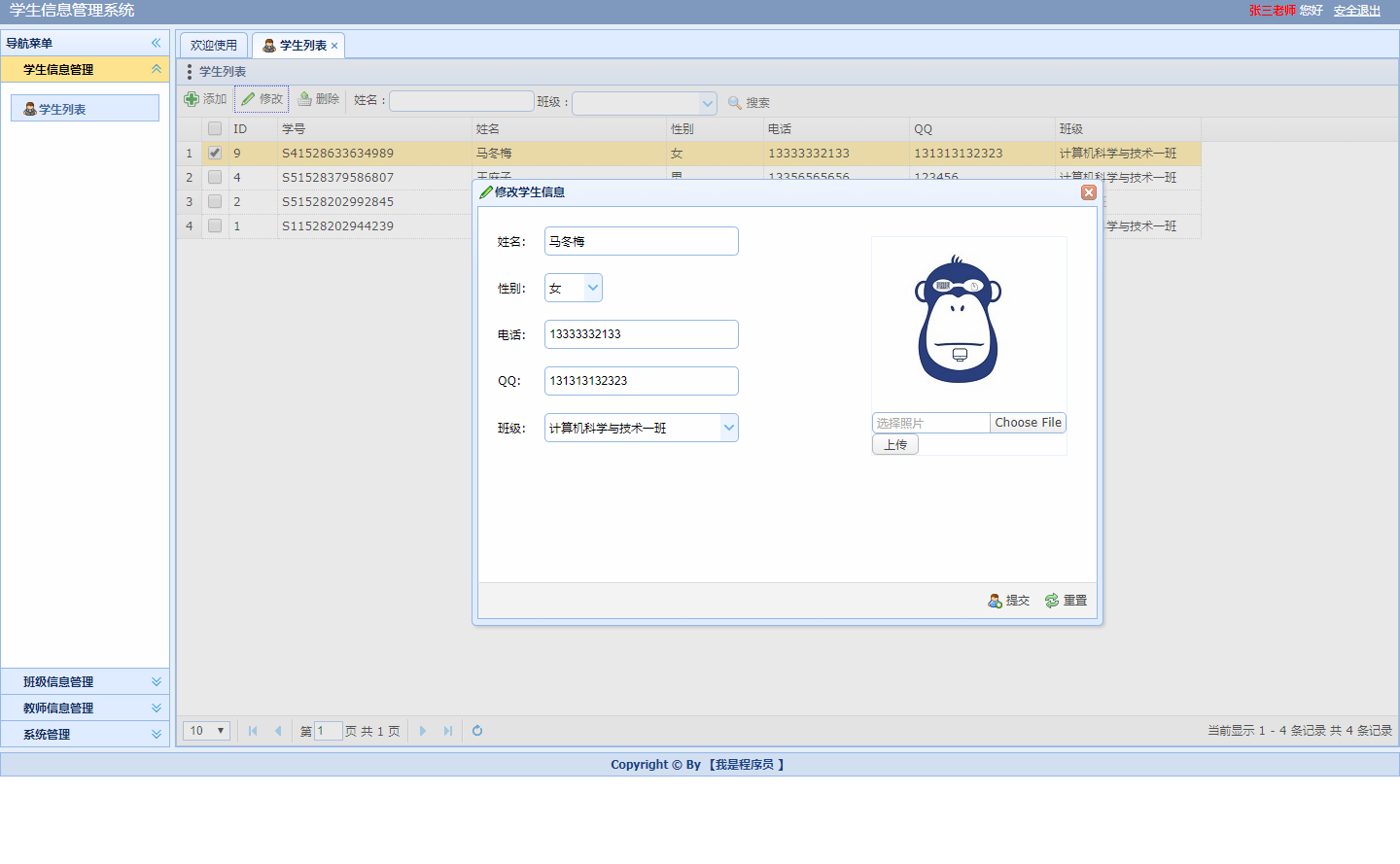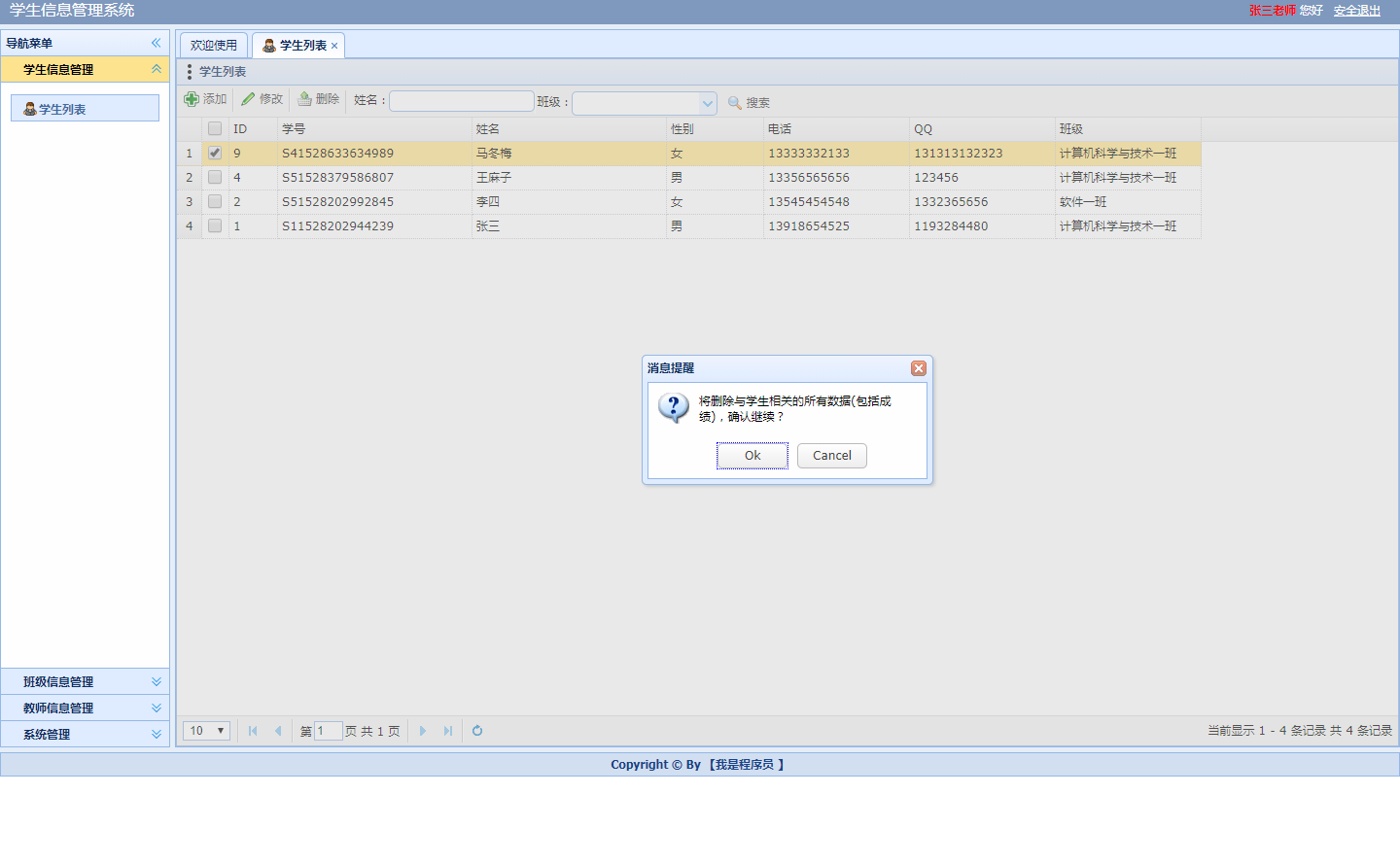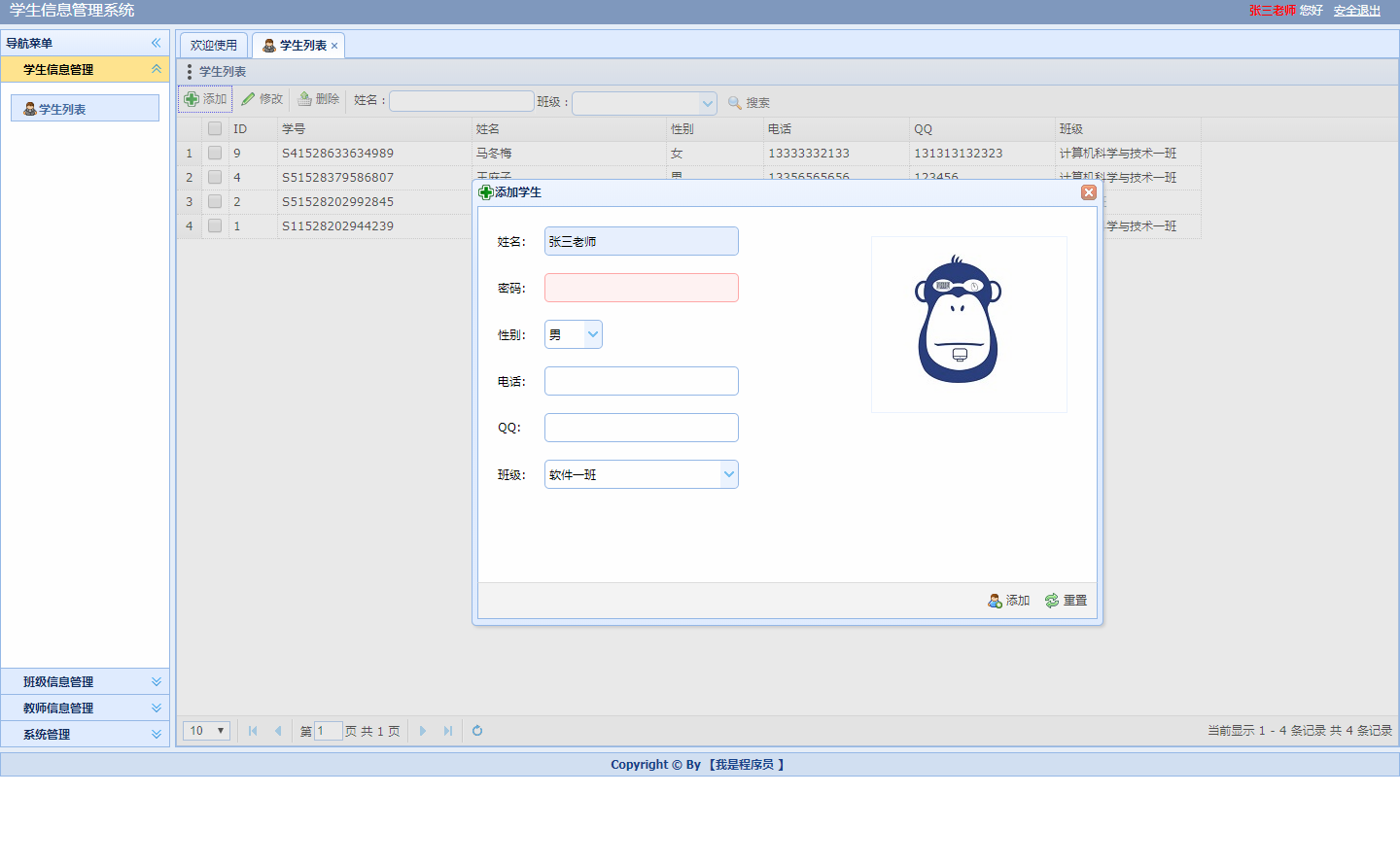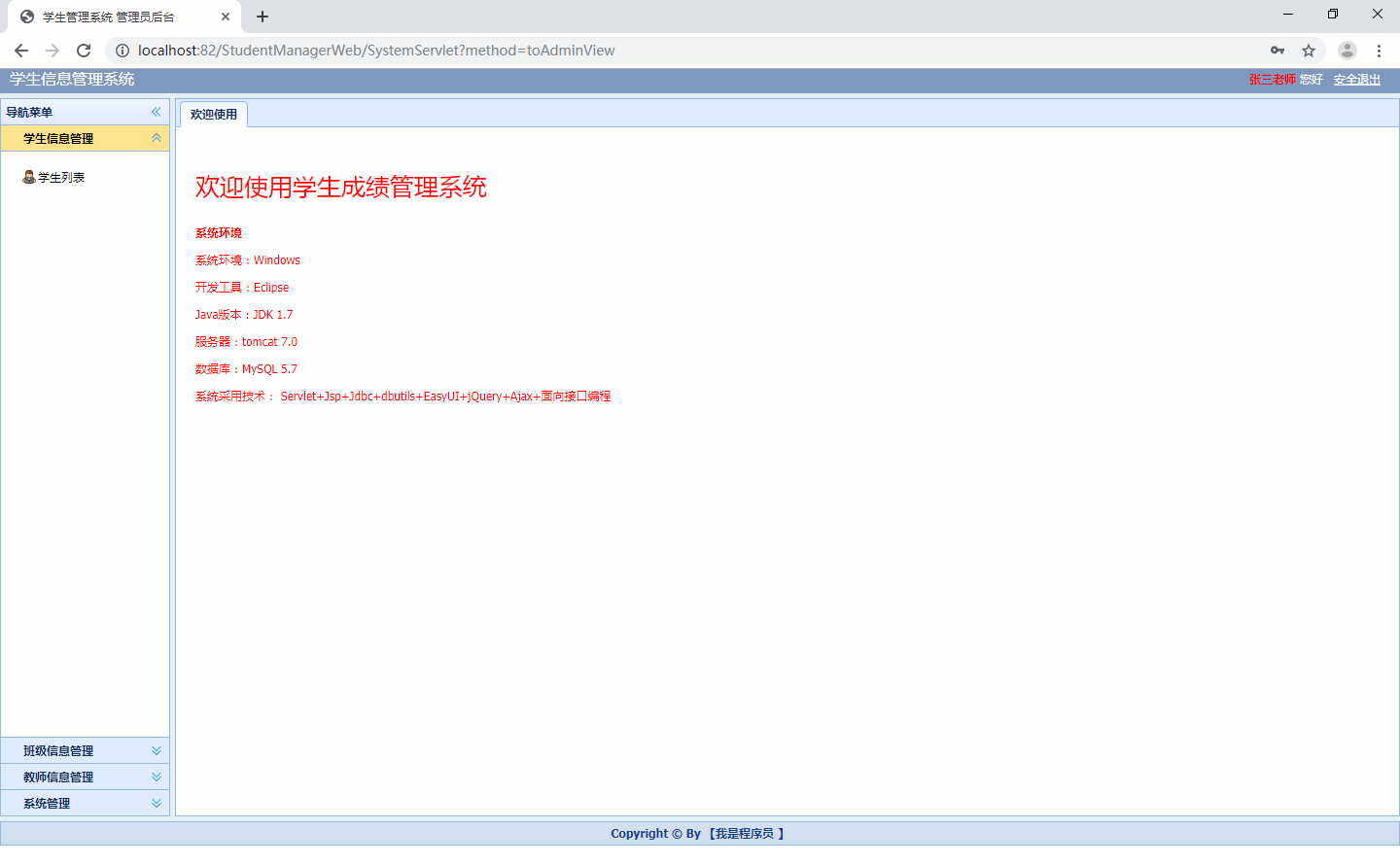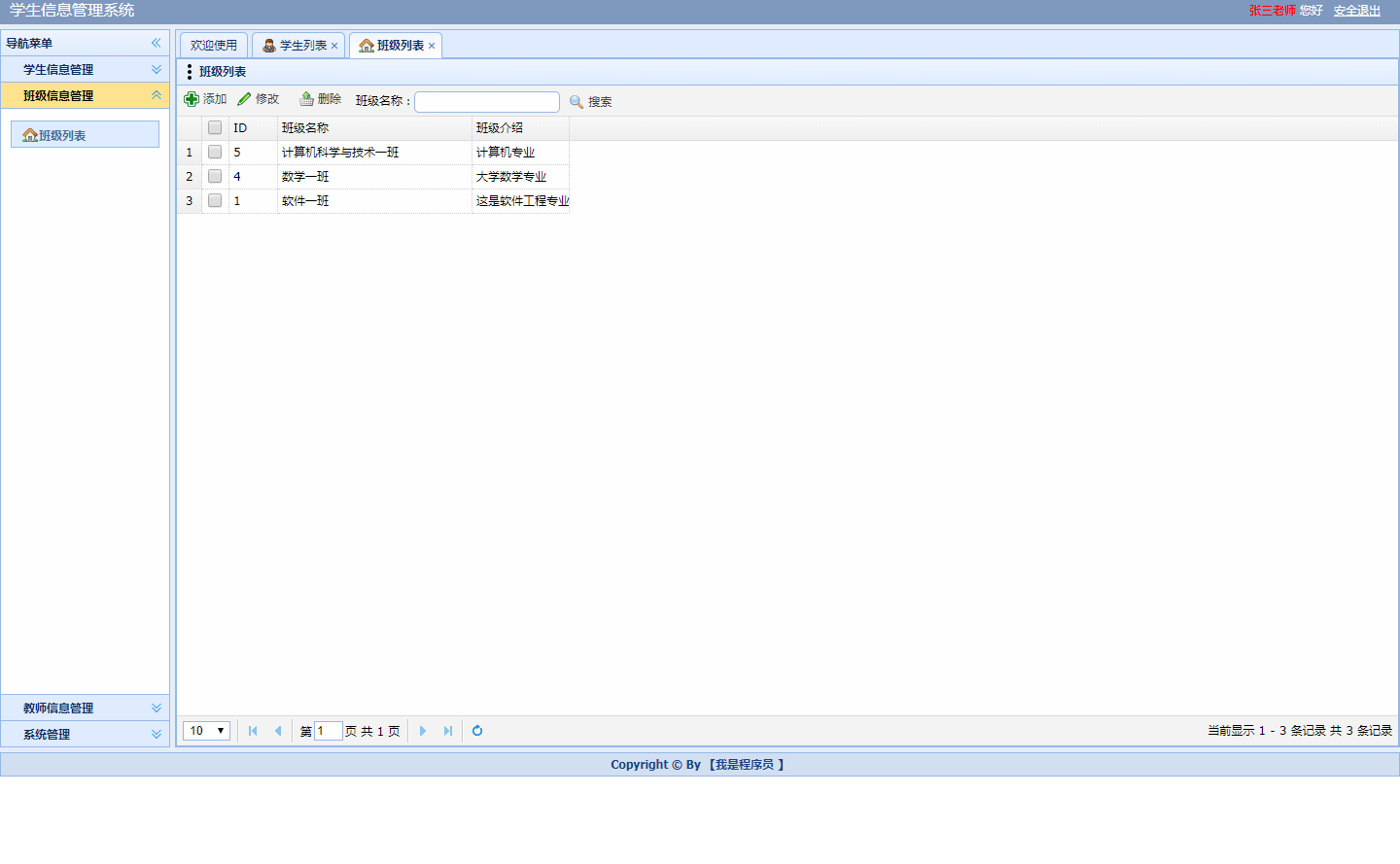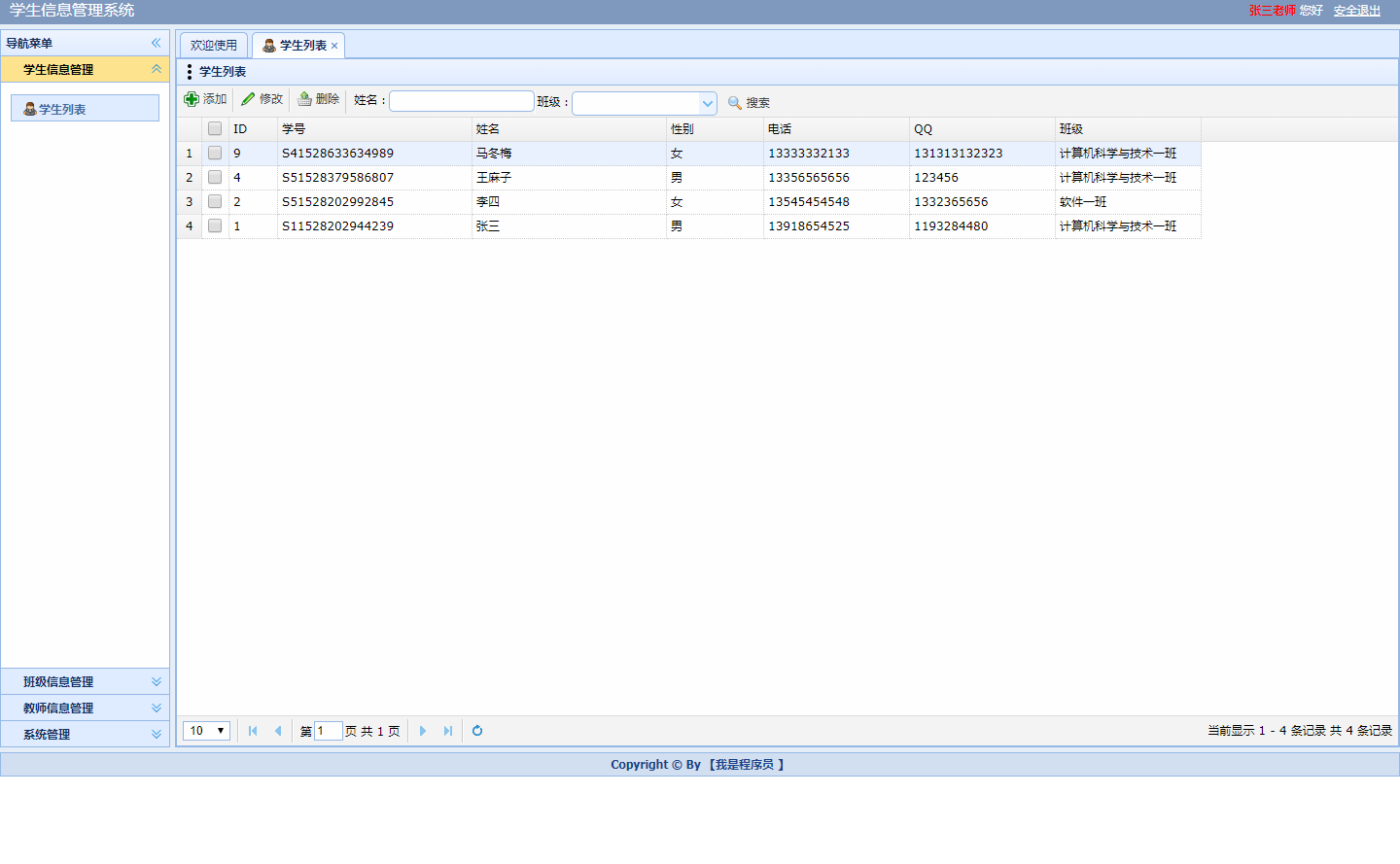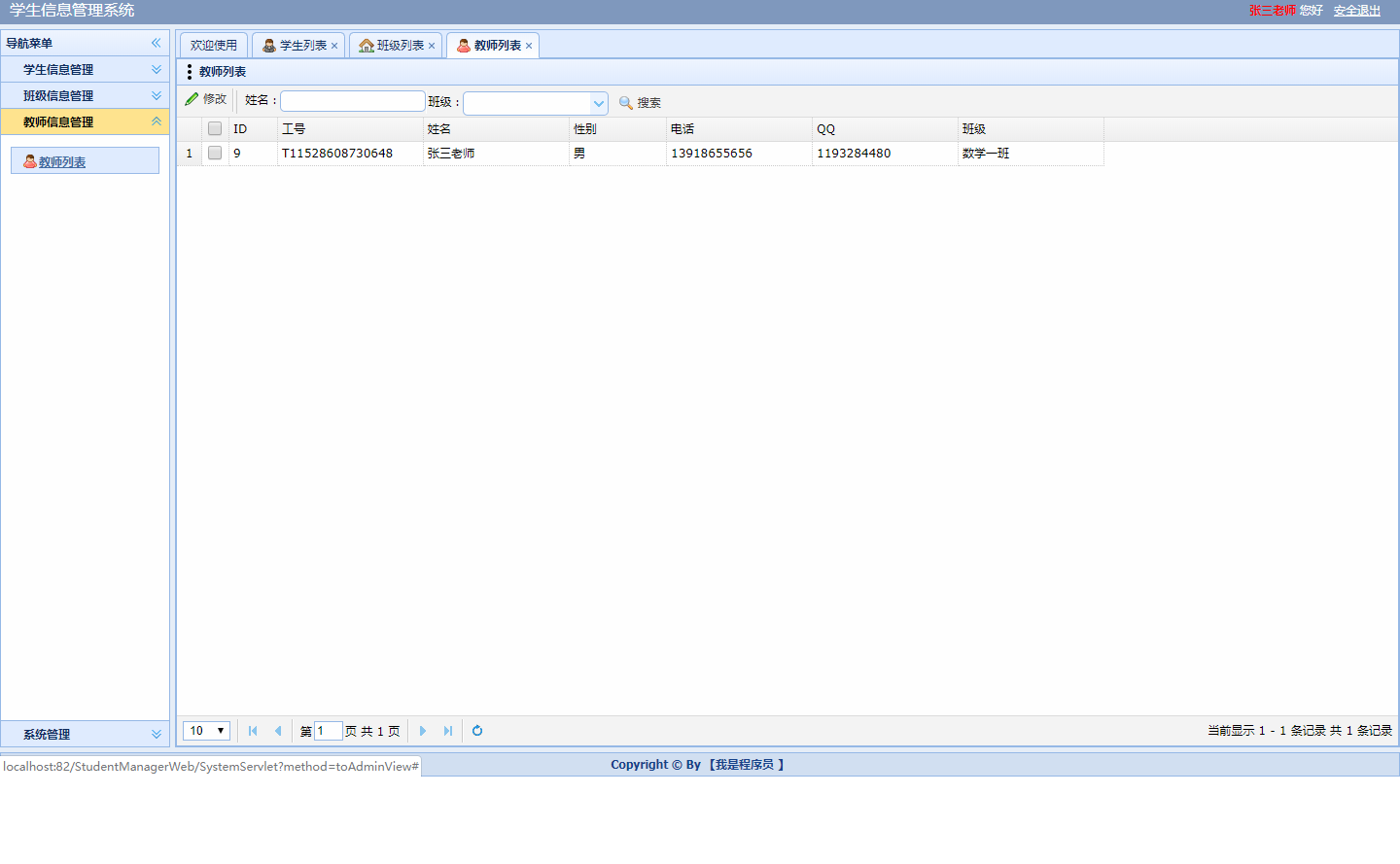### 4.管理员页面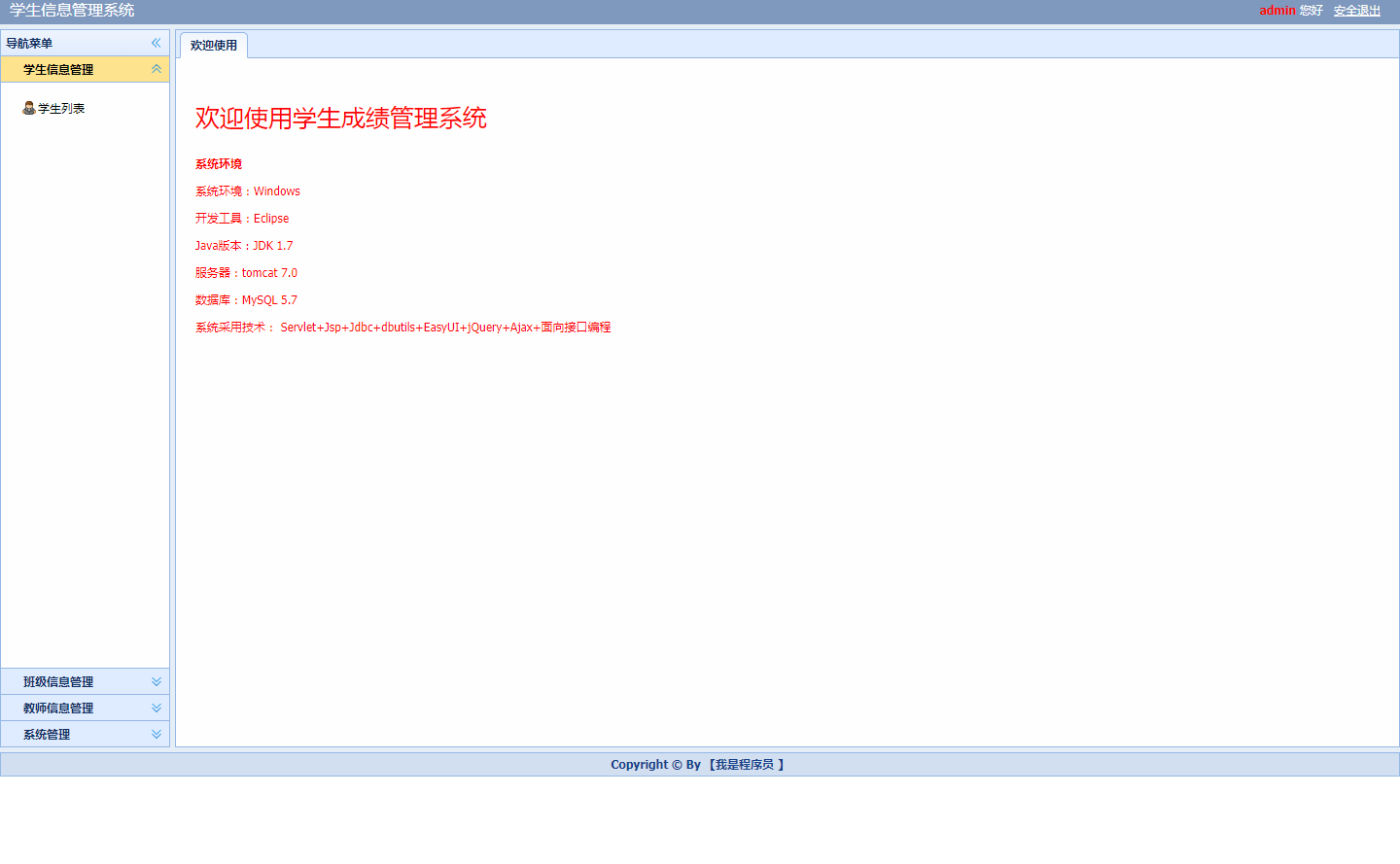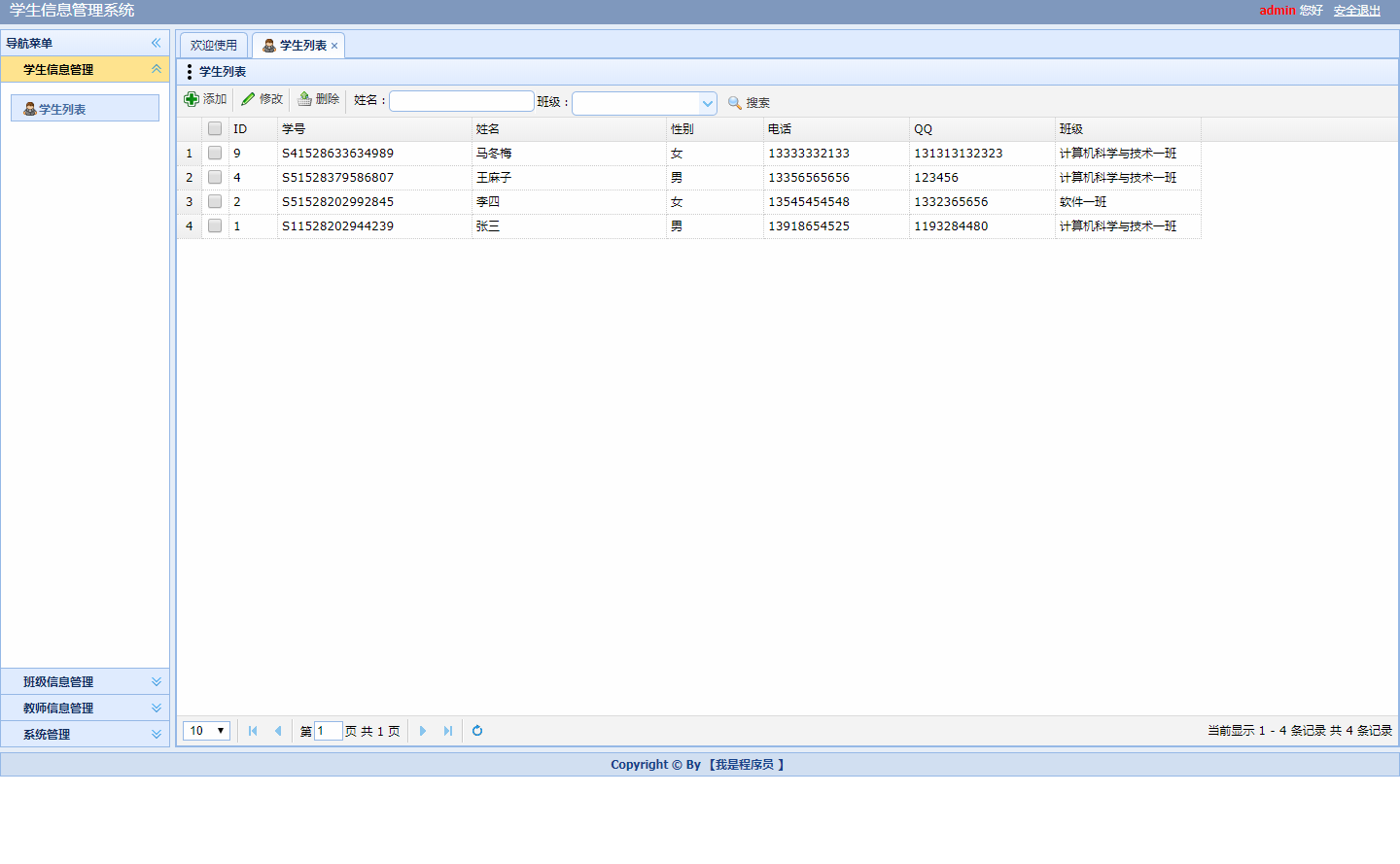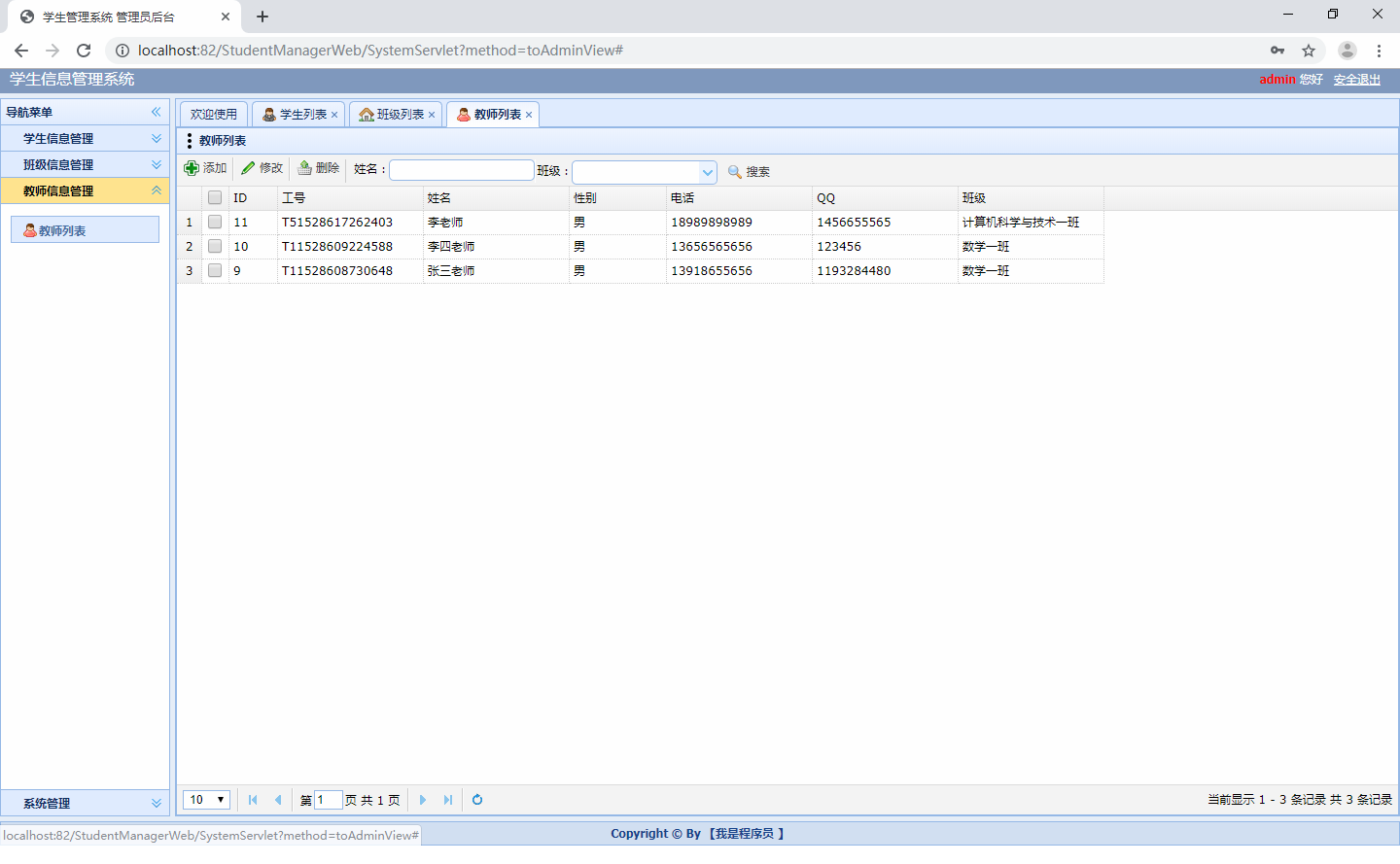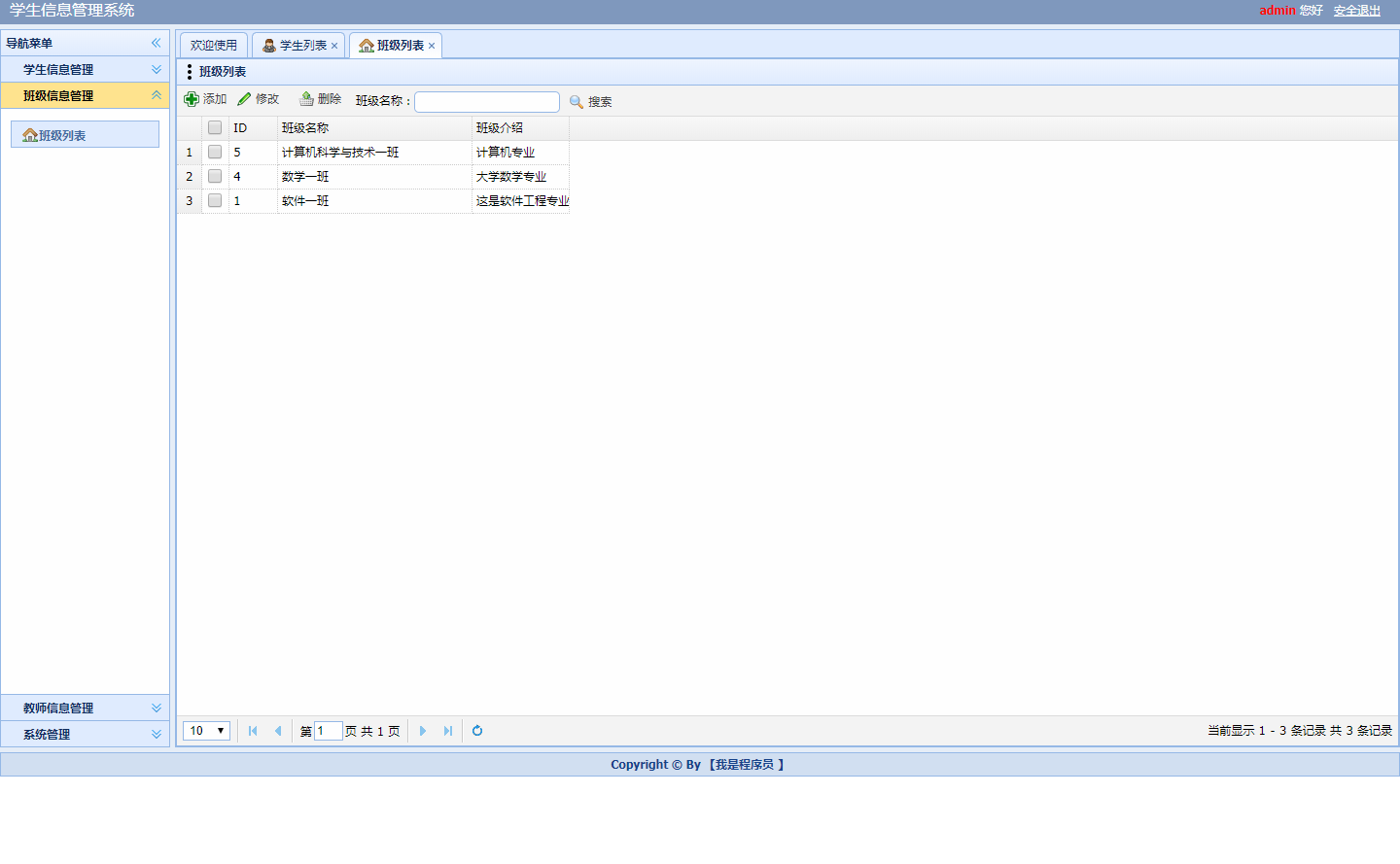ssm学生成绩管理系统

# 该源码已收录【java项目源码】中，需要的请自行关注下载展开全文ssm...